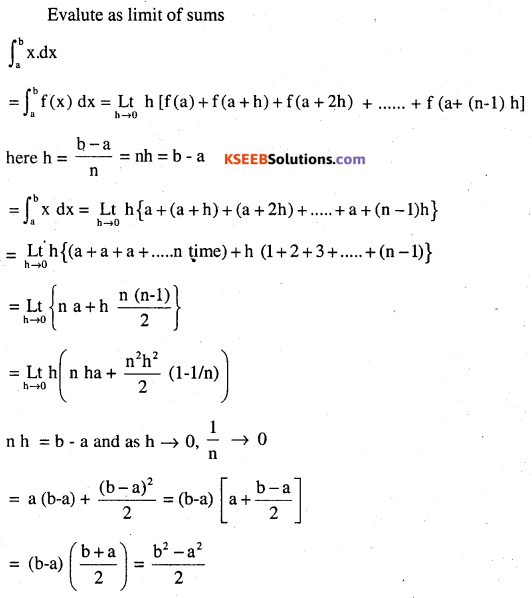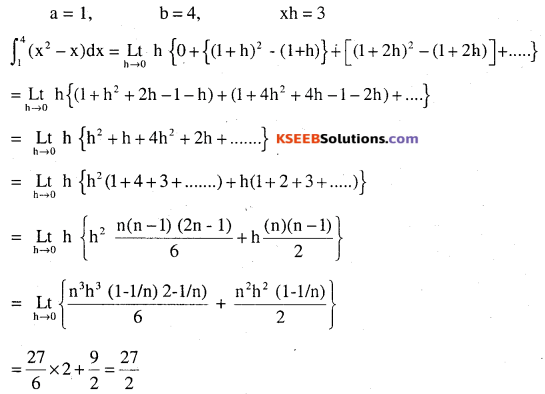# 2nd PUC Maths Question Bank Chapter 7 Integrals Ex 7.8

Students can Download Maths Chapter 7 Integrals Ex 7.8 Questions and Answers, Notes Pdf, 2nd PUC Maths Question Bank with Answers helps you to revise the complete Karnataka State Board Syllabus and score more marks in your examinations.

## Karnataka 2nd PUC Maths Question Bank Chapter 7 Integrals Ex 7.8

### 2nd PUC Maths Integrals NCERT Text Book Questions and Answers Ex 7.8

Evaluate the following definite integrals as limit of sums

Question 1.
$$\int_{a}^{b} x d x$$Question 2.
$$\int_{0}^{5}(x+1) d x$$Question 3.
$$\int_{2}^{3} x^{2} d x$$Question 4.
$$\int_{1}^{4}\left(x^{2}-x\right) d x$$Question 5.
$$\int_{-1}^{1} e^{x} d x$$$$\int_{0}^{4}\left(x+e^{2 x}\right) d x$$